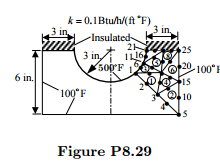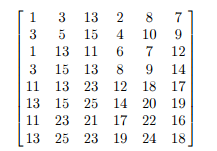# Formulate The Finite Element Analysis Information To Determine The Temperature Distr 2212693

Formulate the finite element analysis information to determine the temperature distribution in the molded asbestos insulation shown in Fig. P8.29. Use the symmetry to identify a computational domain and give the specified boundary conditions at the nodes of the mesh. What is the connectivity of matrix for the mesh shown?sol\$

The computational domain is shown in Figure P8.29 (the finite element mesh part). The heat flux is zero along the insulated boundary and line of symmetry. Nodes 5, 10, 15, 20, and 25 have a specified temperature of 100◦F, and nodes 1, 6, 11, 16, and 21 have a specified temperature of 500◦ F. The assembled coefficient matrix is of order 25 × 25, and the condensed coefficient matrix is of order 15 × 15. The connectivity matrix is given by